Organic chemistry 2009 VCE Cinnamic acid is an organic substance that partly contributes to the flavour of oil of cinnamon. A structure of cinnamic acid is given below.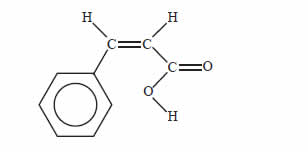Which of the following reagents would you expect to react with cinnamic acid under the conditions given below?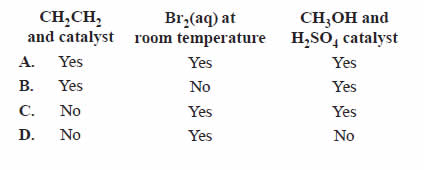SolutionA product derived from palm tree oil is used as an alternative fuel in diesel engines. Palm oil is converted to biodiesel by the following reaction.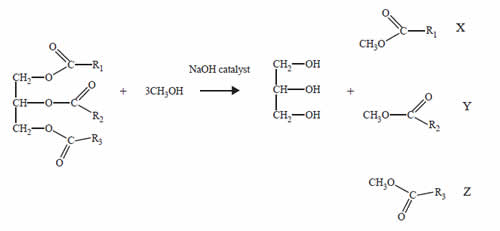Glycerol is separated from the reaction mixture. The mixture of the compounds labelled X, Y and Z is used as palm oil biodiesel. The term that best describes the mixture of compounds in palm oil biodiesel is A. methyl esters. B. carbohydrates. C. carboxylic acids. D. monoglycerides. Solution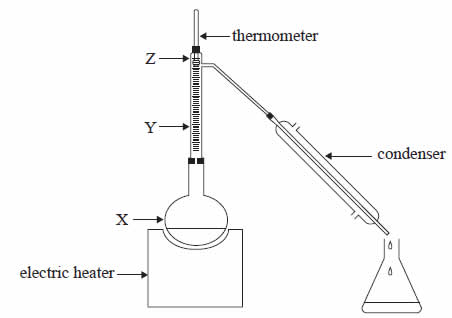A liquid mixture of 50% ethanol and 50% water was distilled in the apparatus shown above. The boiling point of ethanol is 78°C and that of water is 100°C. As the mixture was heated the temperature shown by the thermometer initially rose but then remained constant at 78°C for some time. Which one of the following statements about percentage of ethanol in the vapours shown at points X, Y and Z, when the temperature is at a constant 78°C, is true? A. The percentage of ethanol in the vapours at X is equal to 50%. B. The percentages of ethanol in the vapours increase in order at positions X, Y and Z. C. The percentages of ethanol in the vapours at Y and Z are equal but greater than at X. D. The percentages of ethanol in the vapours at X, Y and Z are equal but greater than 50%. SolutionMyrcene is a naturally occurring compound found in the leaves of bay trees. It is known to be a polyunsaturated hydrocarbon. It can react with hydrogen to produce a saturated hydrocarbon. In a laboratory investigation, a 1.00 g sample of pure myrcene fully reacted with exactly 510 mL of hydrogen gas measured at 20.0°C and 105.0 kPa. In this reaction, myrcene was converted to a saturated alkane with a molecular formula C10H22. a. What type of reaction has occurred between the myrcene and hydrogen? Solutionb. Calculate the amount, in moles, of hydrogen reacting. Solutionc. Calculate the mass of C10H22. produced in the reaction. Solutiond. Determine the number of double bonds in each molecule of myrcene. SolutionMany esters are used as flavouring agents in food. The structure of the ester used in raspberry flavouring is provided below.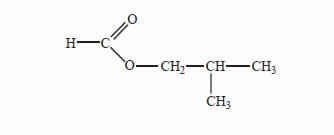Give the names of two carbon compounds that can be used to synthesise this ester. SolutionGlucose can combine with fructose to form the disaccharide, sucrose. Sucrose (molar mass 342 g mol–1) is highly soluble in water. However, 1-butanol, a much smaller molecule (molar mass 74 g mol–1), is much less soluble in water. Explain, in terms of the structure of these two molecules, why this is the case. The structure of glucose and fructose was given on the data sheet. Solution